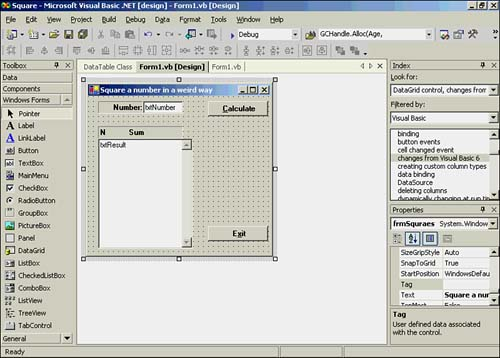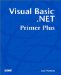# Using a For - Next Loop to Implement an Algorithm

### Using a For - Next Loop to Implement an Algorithm

Algorithm? An algorithm is nothing more than blueprint, or recipe, of how a particular programming problem is going to be resolved. In this section, I'm going to describe to you something I observed , and then let you try to develop an algorithm to solve the problem. After all, this is the way programs are developed in the real world. That is, the potential user describes what he wants and expects you to develop the algorithm(s) necessary to implement it.

Quite by accident , I discovered that for a given number, N, the sum of N odd positive integers is equal to the square of N.

Write a program that demonstrates this mathematical result. Now, read the sentence a few times to let it sink in. Okay, now let's try to make sense of what it says.

First, let's pick a number and see whether the description as given is correct. Let's try N equals 3. Next, it says that if we add up N odd positive integers, the sum of those integers should equal the square of the number. So, we have

` N = 3  Sum = 1 + 3 + 5     (Since N equals 3, then 1, 3, and 5              are the 3 positive integers.) Sum = 9 `

Hmmm. It seems to work; N 2 does equal 9. Let's try it for N equal to 5:

` N = 5  Sum = 1 + 3 + 5 + 7 + 9 Sum = 25 `

Weird, but it works. Visually, I can explain it. For example, put a single floor tile on the floor and it forms a square. Add three more tiles and you can still form a square. Add another five tiles and you can still create a larger square. I don't have a clue how to state this mathematically, however. Further, because it's never come up at a cocktail party, maybe I don't even care to know!

Now let's design a program that implements the algorithm.

First, we need a text box that accepts the number that we want to square. (This would be our Input step from Chapter 3, "Thinking About Programs.") We'll call it txtNumber . We also need some way to display the results (the Output step from Chapter 3). However, rather than just displaying the square of the number, perhaps we should show how the number was derived. After all, we could cheat and just square the number and the user wouldn't know the difference.

Okay, that's good for a start. Create a new project and call it Square. Place the components on the form in a manner similar to that shown in Figure 12.3.

##### Figure 12.3. Component layout for the Square project.The Calculate button is named btnCalc and the exit button is btnExit . To be able to "stretch" the txtResult text box, you must first set its Multiline property to True . We've also added vertical scrollbars to the text box.

Now all we have to do is think about how we want to implement the algorithm in code. We definitely need a loop counter and a variable to keep the sum of the odd integers. Because the integer value increases by 2 during each iteration through the loop, we need a variable to track that value, too. Listing 12.3 presents the code.

##### Listing 12.3 Code for the btnCalc Click Event
` Private Sub btnCalc_Click(ByVal sender As System.Object, ByVal e As _              System.EventArgs) Handles btnCalc.Click   Dim Number, Sum, i, MyInteger As Integer   Dim Newline As String   Newline = Chr(13) & Chr(10)   Sum = 0   MyInteger = 1   Number = CInt(txtNumber.Text)   txtResult.Text = ""   For i = 1 To Number    Sum += MyInteger    txtResult.Text += CStr(i) & "  " & CStr(Sum) & Newline    MyInteger += 2   Next  End Sub `

#### An Alternative Syntax to Define Variables

Notice how we've defined the variables in this program. In previous programs, we've defined the variables as

` Dim Number As Integer, Sum As Integer, i As Integer, MyInteger As Integer `

specifying the data type for each variable as it is defined. However, Visual Basic .NET enables us to use the format shown in Listing 12.3 with the same result, but using a short syntax notation.

Most of the rest of the code in Listing 12.3 should look familiar to you, so let's concentrate on the For loop. Variable i serves as our loop counter and Number dictates how many iterations through the loop are to be made. Sum holds the running total for the loop, and MyInteger is used to hold the integer value as the loop executes. Notice how MyInteger is initialized to 1 outside the loop.

#### Code Walk-Through

Now let's do a walk-through of the code. A code walk-through is when you take a piece of paper and, without running the program, execute the code in you mind, line by line. You can jot down values of key variables as you mentally run the program. For our walk-through, we'll assume the number to square is 3. This means that the value of Number is 3. Use Listing 12.3 as you read this section.

On the first pass through the loop, Sum is assigned the value of MyInteger in the first statement of the For loop statement block. Therefore, Sum should equal 1 when i equals 1 . The next statement displays i and Sum , which now should both equal 1. The third statement increments MyInteger by 2, making its value 3 . We now hit the Next keyword, which increments i by 1. Because i is less than Number , Visual Basic .NET sends control back to the first statement in the statement block.

MyInteger is added to Sum . Prior to the addition, Sum equaled 1 . Because MyInteger is now 3 , Sum equals 4 after the statement is executed. The loop counter i is 2 at this point and the values are displayed in the text box. MyInteger now has 2 added to its current value of 3, to yield its new total of 5. The Next statement bumps i by 1. Because i is still less than Number , control goes back up to the first statement in the block.

Again, MyInteger is added to Sum . Sum currently equals 4 and MyInteger equals 5 . After the addition, Sum equals 9 . The loop counter i is now 3. The following statement displays the data. MyInteger is again incremented by 2, making its value 7 .

The Next statement increments i to 4 . However, this time i is now greater than Number . Therefore, Next does not send control back for another pass. Instead, control passes to the statement following Next in the program. Because the next program statement is End Sub , the program is finished processing the Click event.

We've finished our code walk-through, and Sum equals 9 and Number equals 3 . It appears our code does the job. You should compile and run the program to verify that the code works as advertised.

#### Mental Itching

The code in Listing 12.3 performs as advertised, but an itch in the back of your mind might need to be scratched. Sum and MyInteger seem to be doing about the same thing in our program. Another thing that bothers us is that we know we can make the For loop increment by any value we want by simply adding a Step keyword to the statement.

Let's modify the code in the For loop to that shown in the following code fragment:

` For i = 1 To (Number * 2) Step 2   Sum += i  txtResult.Text += CStr(MyInteger) & " " & CStr(Sum) & Newline MyInteger += 1 Next `

First of all, notice that we have added a Step keyword at the end of the For statement. The Step keyword tells the Next statement how much to increment the loop counter. If Step isn't specified, the default increment is 1. In our modified code, we're telling Visual Basic .NET to increment i by 2 on each pass through the loop. However, because we're incrementing i by 2 on each pass through the loop, we need to double the terminating value of the loop, which is Number . If we didn't do this, we wouldn't make the required number of passes through the loop.

The statement

` Sum += i `

simply adds i to Sum . That is, i can assume only odd integer values now because we're adding 2 to i on each pass. Therefore, if i starts at 1, its values become 1 , 3 , and 5 for the three passes through the loop. Again, this is because we overrode the default Step value of 1 used by the For loop and made it 2 .

The statement to display the output in the txtResult text box is the same, except that we're displaying MyInteger instead of i for the first number displayed. Note that we are incrementing MyInteger by 1 now, so we can use it in the display to show which pass we're making through the loop. By the way, the actual assembly language code for incrementing a variable by 1 is usually one of the fastest instructions a CPU can do. Therefore, the increment on MyInteger will be extremely fast.

If you make the code changes and compile the program, you'll see that the program does calculate the square correctly.

#### Which Version Is Better?

Okay, so what do you think of our modifications? Which version of the program do you like better, and why? Think about your decision for a moment.

Personally, I don't like the second version all that much. The required multiplication of Number by 2 in the For statement confuses the intent of the algorithm. The Step instruction is also a distraction. Also, if there is any performance improvement, it's going to be very minor. As a result, I would probably scrap this last effort and stick with our first version of the program.

Some of you are probably a tad miffed at me right now because I made you read a few extra pages and maybe unnecessarily killed an extra tree or two. Well, not really. The process shown here is how things work in the real world. Sometimes your first try at something is the best, but most of the time a little thought will improve it  . In this case, it didn't. I apologize. Sometimes the second effort has more warts than the first and, when that happens, you need to admit it and change the code back to the way it was. However, rethinking a solution is almost always a good thing, so even this step backward is a positive learning experience.

 Jack Purdum, "Pattern Matching in C," Computer Language , November 1987. The article shows how minor algorithm changes produced an almost tenfold speed improvement.

Also, one of the primary reasons I think the first effort is better is because the intent of the code is more clearly understood in the first program. Although the second effort may exhibit more cleverly crafted code, clever code isn't necessarily good code. This is especially true when the clever code is difficult to understand. A major component of software development cost is debugging and, ceteris paribus (all other things being equal), clever code is harder to debug than simple, straightforward code. Good program managers abide by the KISS principle (Keep It Simple, Stupid) because they know it helps keep development costs down. If you can't resist being clever, make sure that you add enough comments to the code to demystify it.Visual Basic .NET Primer Plus
ISBN: 0672324857
EAN: 2147483647
Year: 2003
Pages: 238
Authors: Jack Purdum

Similar book on Amazon